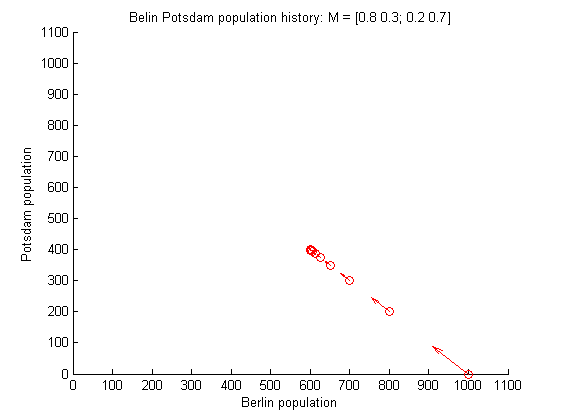## 2012-12-23

### Authors in a Markov matrix: Which author do people find most inspiring? (21)

Here we don't want to consider what is the result of applying a matrix to a vector, but we would like to consider what the property of a matrix. How all the vectors behave by a matrix, not only one specific vector. Because the combination of inhabitants of Berlin and Potsdam. Even the total inhabitants is fixed, i.e., 1,000,000 then, we can make 1,000,001 different vectors. We can also change the total inhabitants to any number. However, you have already seen, this matrix can be described two numbers (= one vector). This is the reason eigenanalysis is an important idea in linear algebra. Because you can only think just two numbers, instead of millions in this example.

When Berlin and Potsdam's inhabitants started by any number, enough years later, the inhabitants becomes specific numbers.

The vector of inhabitants of Berlin 600, Potsdam 400 is a special vector of this matrix and therefore, it has a name. It is called an eigenvector. Figure 12 shows this vector on Figure 13. You see all the initial state converges on the eigenvector in Figure 13.Figure 12: Population history of Berlin and Potsdam.Figure 13: Population history of Berlin and Potsdam with $y =\frac{400}{600}x$ line.
Eigenvector $$\vec{x}$$ is a special vector regarding matrix $$M$$, such that
\begin{eqnarray*}
M\vec{x} &=& \lambda \vec{x}.
\end{eqnarray*}
Where the $$\lambda$$ is a scalar value. This is neither a matrix, not a vector, just a number. This $$\lambda$$ also has a name, eigenvalue.

In this example,  $$\lambda = 1$$ as you see in the following.
\begin{eqnarray*} M \left[ \begin{array}{c} 600 \\ 400 \\ \end{array} \right] &=& \left[ \begin{array}{c} 600 \\ 400 \\ \end{array} \right] \end{eqnarray*}
This vector can be multiplied by any scalar number.
\begin{eqnarray*} M \left[ \begin{array}{c} 6 \\ 4 \\ \end{array} \right] &=& \left[ \begin{array}{c} 6 \\ 4 \\ \end{array} \right] \end{eqnarray*}
The eigenvalue is also an incredible number to me. If you see the above equation, it looks like matrix $$M$$ is equal to one number. In this example, the matrix size is 2x2, but, even 1000x1000 matrix, the eigenvalue is a scalar. That means the essence of this complex matrix is squeezed to a scalar number. I have a problem to understand 1000 dimensional matrix, but, I have some feeling if it is just one number.

Here we have forgot a vector and get some property of the matrix. Let's see what happens if we apply the matrix to the matrix instead of a vector.

octave:2> M
0.80000   0.30000
0.20000   0.70000
octave:3> M^2
0.70000   0.45000
0.30000   0.55000
octave:4> M^3
0.65000   0.52500
0.35000   0.47500
octave:5> M^5
0.61250   0.58125
0.38750   0.41875
octave:6> M^10
0.60039   0.59941
0.39961   0.40059
octave:7> M^100
0.60000   0.60000
0.40000   0.40000

The numbers in the last result is familiar.

In this article, I don't explain the algorithm to compute the eigenvectors and eigenvalues. Curious reader can refer . We apply the matrix $$M$$ multiple times and see it converges. But this only happens when the largest eigenvalue of this matrix is 1. Not all matrices has the largest eigenvalue 1. However, a Markov matrix has the largest eigenvalue 1. The curious readers can found why a Markov matrix has the largest eigenvalue 1 in Appendix B.

You can often find the story of population change and eigenvector, since this is a kind of standard example. Though I found the book  is interesting. I also like Shiga's book  about eigen problem. My favorite linear algebra book is .

## References

 Yoshio Kimura, Fun of linear algebra for freshman (Daigaku ichinensei no tameno omosiro senkeidaisuu,'' Gendai Suugakusha, 1993

 Kouji Shiga, 30 Lectures of eigen problem (Koyuuchi mondai 30 kou,'' Asakura shoten, 1991

 Gilbert Strang, Introduction to Linear Algebra, 4th Edition,'' Wellesley-Cambridge Press,
2009